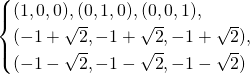# A gentle (and short) introduction to Gröbner Bases

Taken from my report for a Computer Algebra course.

## Motivation

We know there are plenty of methods to solve a system of linear equations (to name a few: Gauss elimination, QR or LU factorization). In fact, it is straightforward to check whether a linear system has any solutions, and if it does, how many of them there are. But what if the system is made of non-linear equations? The invention of Groebner bases and the field of computational algebra came up to answer these questions.

In this text we will recap the theory behind single-variable polynomials and extend it to multiple-variable ones, ultimately getting to the definition of Groebner bases.

In some cases, the transition from one to multiple variables is smooth and pretty much an extension of the simple case (for example for the Greatest Common Divisor algorithm). In other cases, however, there are conceptual jumps to be made. To give an example, single variable polynomials have always a finite number of roots, while this does not hold for multivariable polynomials. Intuitively, the reason is that a polynomial in one variable describes a curve in the plane, which can only intersect the x-axis a discrete and finite number of times. On the other hand, a multivariate polynomial describes a surface in space, which will always intersect the 0-plane in a continuum of points.

## Preliminaries

All throughout these notes, it will be important to have in mind some basic algebra definitions.

To begin with, we ask what is the most basic (but useful) structure we can put on a set. We ask, for example, given the set of natural numbers, what do we need to do to allow basic manipulation (i.e. summation)? This leads us to the definition of group.

DEF 1: A group is made of a set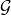with one binary operation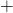such that:

• The operation is closed: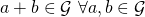• The operation is associative: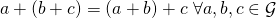• The operationhas an identity element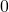s.t.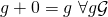• Each element has an inverse element: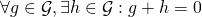A group is usually denoted with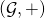.

Then, the notion of group can be made richer and more complex: first into that of ring, then into that of field.

DEF 2: A ring is a group with an extra operation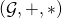which sastisfies the following properties:

• The operationis commutative: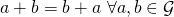• The operationis closed: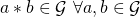• The operationhas an identity elements.t.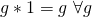• The operationis associative: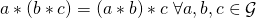• The operationis distributive with respect toDEF. 3: A field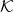is a ring in which all elements have an inverse with respect to the operation.

All throughout these notes, the symbolwill denote a field.

DEF 4: A monomial is a product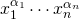, with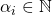. Its degree is the sum of the exponents.

DEF 5: A polynomial is a linear combinations of monomials.

We conclude by noting that the space of polynomials with coefficients taken from a fieldmakes a ring, denoted with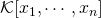.

## Affine varieties and ideals

Our first step towards formalizing the theory for non-linear systems is to understand what the space of solutions looks like. As much as we know that linear spaces are the solutions spaces for linear systems, there is something analogous for non-linear systems, and that is affine varieties.

DEF 6: Given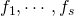polynomials in, the affine variety over them is the set of their common roots: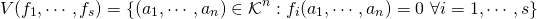EX 1: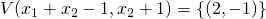When working with rings, as it is our case, the notion of ideal is important. The reason for its importance is that ideals turn out to be kernels of ring homomorphisms — or, in other words, that they are the “good sets” that can be used to take ring quotients.

DEF 7: An ideal is a subset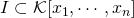such that:

•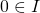• it is closed w.r.t +: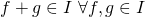• it is closed w.r.t * for elements in the ring: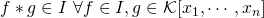Given some elements of a ring, we might wonder what is the way to build an ideal (the smallest) that would contain them.

DEF 8: Givenpolynomials, the ideal generated by them is the set of combinations with coefficients taken from the ring:Having introduced ideals, we immediately find a result that is linked to our purpose of non-linear systems inspection: a simple way to check if a system has solutions or not.

THEO 1: If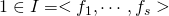, then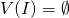.
PROOF: Since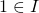, it must be possible to write it as a combination of the form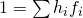. Now, if we suppose that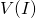is not empty, then one of its pointsis a root of all the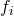. This would mean that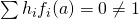, which is absurd.

## Groebner bases

Groebner bases give a computational method for solving non-linear systems of equations through an apt sequence of intersection of ideals. To state its definition, we first need to know what a monomial ordering is. Intuitively, we can think of such an ordering as a way to compare monomials — the technical definition does not add much more concept. Different orderings are possible.

Once we have a way of ordering monomials, it is also possible to define the leading monomial (denoted as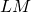) of a given polynomial. For single variable polynomials it is pretty straightforward, but for the multi-variate case we need to define an ordering first (some possible options are: lexicographic, graded lexicographic, graded reverse lexicographic).

DEF 9: Given a monomial ordering, a Groebner basis of an idealw.r.t the ordering is a finite subset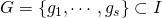s.t.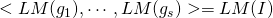.

This basis is a generating set for the ideal, but notice how it depends on the ordering! Finally, it is possible to prove that every ideal has a Groebner basis (Hilbert’s basis theorem).

From here now, the rationale is that, given a system of polynomial equations, we can see the polynomials as generators of some ideal. That ideal will have a Groebner basis, and there is an algorithm to build one (Buchberger algorithm). From there, apt ideal operations will allow to solve the system by eliminating the variables.

We now describe this elimination algorithm with an example:

(1)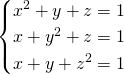Given the ideal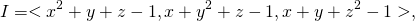then a Groebner basis with respect to the (lexicographical order) is

(2)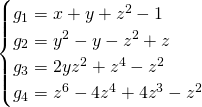which can be used to compute the solutions of the initial system (1).

To do so, first consider the ideal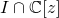, which practically corresponds to all polynomials inwhere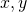are not present. In our case, we are left only with one element from the basis which only involve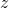: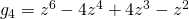. The roots of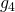are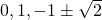.

The values forcan then be used to find the possible values forusing polynomial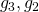, which only involve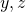. Finally, once possible values forare known, they can be used to find the corresponding values forthrough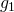.

This example will yield the following solutions:

(3)